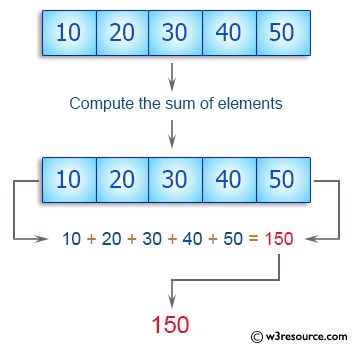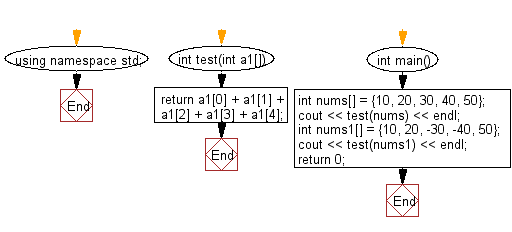﻿ C++ : Compute the sum of the elements of a array of integers# C++ Exercises: Compute the sum of the elements of a given array of integers

## C++ Basic Algorithm: Exercise-84 with Solution

Write a C++ program to compute the sum of the elements of a given array of integers.

Sample Solution:

C++ Code :

``````#include <iostream>
using namespace std;

int  test(int a1[])
{
return a1 + a1 + a1 + a1 + a1;
}

int main()
{
int nums[] = {10, 20, 30, 40, 50};
cout << test(nums) << endl;
int nums1[] = {10, 20, -30, -40, 50};
cout << test(nums1) << endl;
return 0;
}
``````

Sample Output:

```150
10
```

Pictorial Presentation:Flowchart:C++ Code Editor: